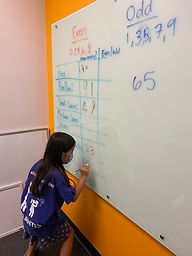## Ms. Briana

### Target 1​

###### Lesson Type:

Continuation

Number Operation

:

Number Classes

Understand that a whole number can be described as even or odd.

###### 1:

Find all the even and odd numbers on a hundred’s chart.

###### 2:

Highlight the pattern that occurs in the number sequence with even and odd numbers (even, odd, even, odd, even…).

###### 3:

Understand that the digit in the ones’ place determines whether a number is even or odd.

###### 4:

Recognize that even numbers have a 0, 2, 4, 6, or 8 in the ones’ place.

###### 5:

Recognize that odd numbers have a 1, 3, 5, 7, or 9 in the ones’ place.

2nd

###### Vocabulary:

Even, Odd and Sequence

Activities:

Students used dice to create different quantities for them to classify as either even or odd.

Students were given a variety of containers with different items and quantities inside. They had to count the contents of each container and classify as them as even or odd. This was done indipendently so that the students could compare their answers and at the end explaining why they classified as they did. (Students who mastered the concept were able to help the other students understand it better)### Home Exploration

###### Guiding Questions:## Absent Students:

### Target 2

:

###### 1:

Identify the pattern that occurs when two even numbers are added.

###### 2:

Identify the pattern that occurs when two odd numbers are added.

###### 3:

Identify the pattern that occurs when an even and odd number are added.

2nd

###### Vocabulary:

Even, Odd, Sum and Sequence

Activities:

Students created their own spinning wheels, one even and one odd.

Students then created addition problems using their own wheels where they added 2 even quantities.

Students then created addition problems using their own wheels where they added 2 odd quantities.

Students then created addition problems using their own wheels where they added 1 even and 1 odd quantity.### Home Exploration

###### Guiding Questions:### Target 3

:

###### 1:

Be introduced to a variety of computation strategies for adding within 1,000 and select a strategy that works best.

###### 2:

Understand that when adding a multi-digit number, each place-value is being added individually to create the new sum (ones are added to ones, tens are added to tens, etc.)

###### 3:

Understand that when adding a multi-digit number, to solve start in the lowest place-value and go left.

###### 4:

Understand that the same process is utilized to add three or more whole numbers that is used to add only two numbers.

###### 5:

Understand how to regroup based on place-value understandings (i.e., ten ones can be composed into one ten – ten tens can be composed into one hundred).

2nd

###### Vocabulary:

Computation, Place-Value and Regroup

Activities:

Students then used base 10 blocks to visually represent regrouping in addition equations (helping understand place value better.

Students pulled numbers out of a box to create addition quantities- alternating between standard form and expanded form to solve the equation.### Home Exploration